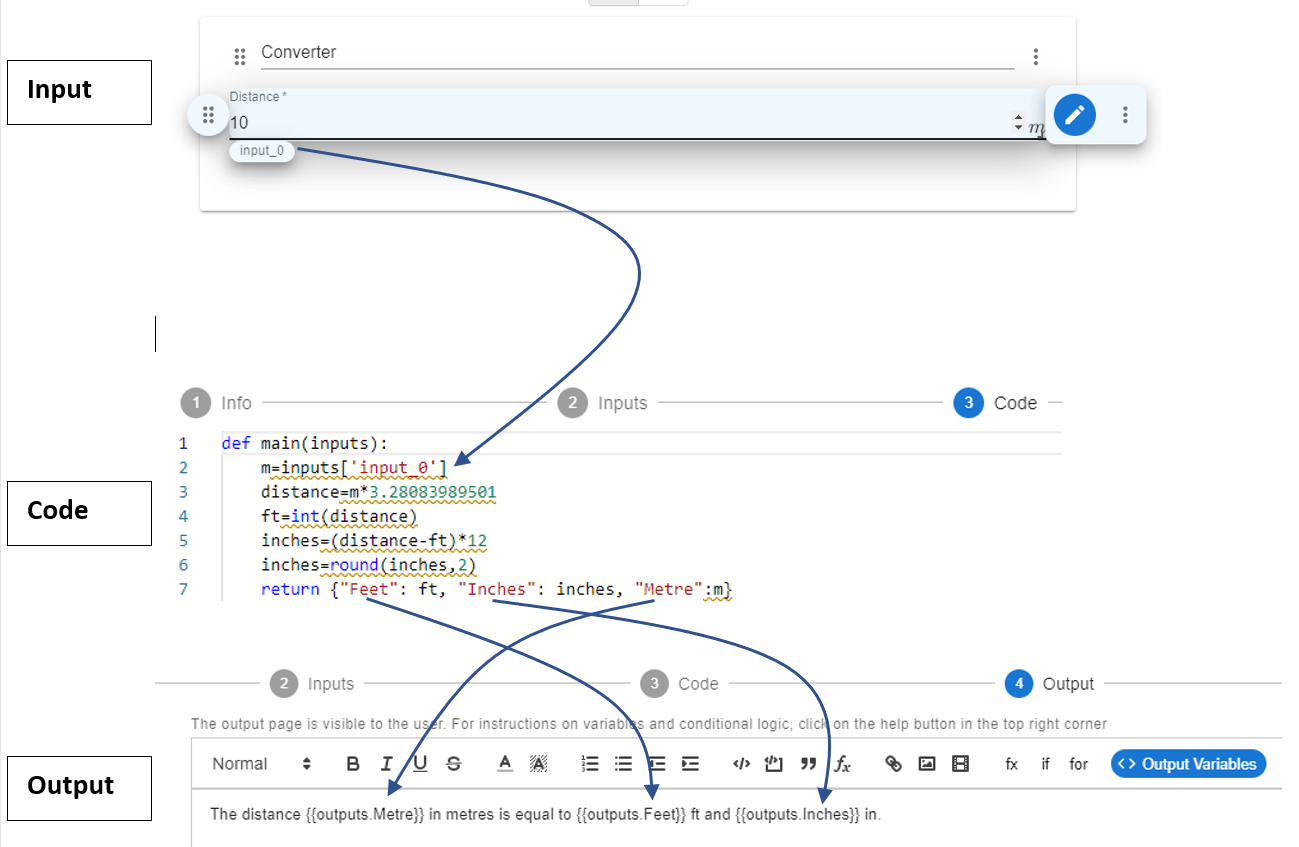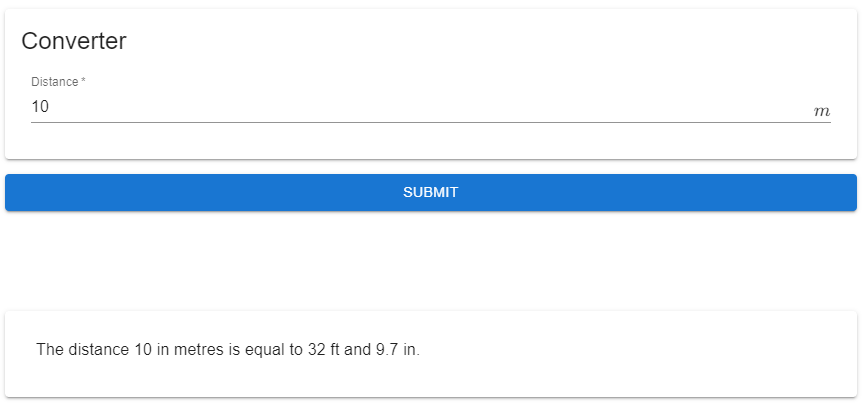# Code Output

## Overview​

The outputs of the main function are available to be inserted in the Output step. The main function should return a dictionary, whose keys can be inserted into the Output editor.

As described in the Distance Converter Example, the following figure shows the overall layout of the inputs, code, and output editors. The main function returns a dictionary with three keys: "Feet", "Inches", and "Metre". The values of each key is the corresponding variables computed in Python: ft, inches, and m. The dictionary keys were then inserted into the output editor as {{outputs.Feet}}, {{outputs.Inches}}, and {{outputs.Metre}}. When a user runs the app, the {{...}} is replaced by the string or numeric value returned from the Python code.Below is an instance of running the app with an input of 10m.## Number Output​

The developer can return any numerical variables defined within the main function. The numerical value can be output as is, or the developer can chose to use any rounding or formatting function to ensure the number has a particular form. As shown in the image above, the variable ft was defined as an integer value while the value inches was defined using the round function. You can also use Python's .format() function for formatting numbers. For example, the following code outputs the variable payment as a dollar value with two decimal places:

def main(inputs):    # ...    # payment = ...    return{"Payment",'\${:,.2f}'.format(payment)}

See the Mortgage Calculator app for an example where the output is in a currency format.

## String Output​

The developer can return string variables from the main function. For example, the following code returns the string variable t, which is input by the user. The .replace() method is used to strip any spaces and create the string variable s, which is also returned by the main function. The two output strings are available in the output editor as: {{ outputs["Original Text"] }} and {{ outputs["Stripped Text"] }}. You can see this app in action here.

def main(inputs):    t = inputs['input_0']    s = t.replace(" ","")    return {"Original Text":t, "Stripped Text":s}

## HTML Output​

As described in Step 4: Output, the developer can design a smart output page using the Output editor, which renders HTML code. The developer can output a string containing HTML, which can then be embedded and displayed in the Output editor. If you are familiar with HTML, you can write a HTML string using HTML tags. Although, many Python libraries have built-in functions to convert to a HTML string, therefore, it is recommended to first search for "convert to HTML" functions in the respective Python libraries. Please review Example 3 which provides the details of outputting a HTML table.

## File Data Output​

The developer can also choose to output file data as a string. The data can then be available for the user to download as a file as described here.